# Integer Type ques of Chemical Kinetics & Nuclear Chemistry, Past year Questions - JEE Advance JEE Notes | EduRev

## JEE : Integer Type ques of Chemical Kinetics & Nuclear Chemistry, Past year Questions - JEE Advance JEE Notes | EduRev

The document Integer Type ques of Chemical Kinetics & Nuclear Chemistry, Past year Questions - JEE Advance JEE Notes | EduRev is a part of the JEE Course Class 12 Chemistry 35 Years JEE Mains &Advance Past yr Paper.
All you need of JEE at this link: JEE

Integer Value Correct Type

1. The total number of a and b particles emitted in the nuclear reaction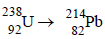Ans: 8

Solution :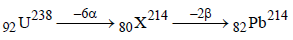Hence total number of particles emitted are 2 +6 = 8

2. The concentration of R in the reaction R → P was measured as a function of time and the following data is obtained: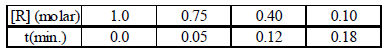The order of reaction is                                     (2010)

Ans: 5

Solution : The integrated form of a zero-order reaction is
[A0] – [At] = k0t ; 1.0 – 0.75 = k0 × 0.05, k0 = 5
Again, 1.0 – 0.4 = k0 × 0.12, k0 = 5

3. The number of neutrons emitted when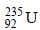undergoes controlled nuclear fission to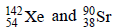is               (2010)

Ans: 3

Solution :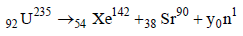235 = 142 + 90 + y Þ y = 3.
The number of neutrons emitted are 3.

4. An organic compound undergoes first-order decomposition. The time taken for its decomposition to 1/8 and 1/10 of its initial concentration are t1/8 and t1/10 respectively. What is the value of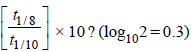(2012)

Ans: 9

Solution :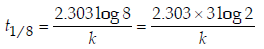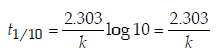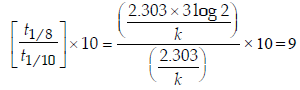5. The periodic table consists of 18 groups. An isotope of copper, on bombardment with protons, undergoes a nuclear reaction yielding element X as shown below. To which
group, element X belongs in the periodic table?                       (2012)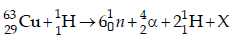Ans:  8

Solution :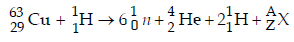Balancing the atomic mass and atomic number
63 + 1 = (6 × 1) + 4 + 2 + A ⇒ A = 52
29 + 1 = (6 × 0) + 2 + 2 + Z ⇒ Z = 26

Thus,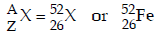Hence, X belongs to group 8 in the periodic table.

6. A closed vessel with rigid walls contains 1 mol  of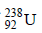and 1 mol of air at 298 K. Considering complete decay ofto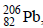, the ratio of the final pressure to the initial pressure of the system at 298 K is                        (JEE Adv. 2015)

Ans: 9

Solution :  Number of moles in gas phase, at start (ni) = 1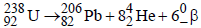Now number of moles in gas phase, after decomposition (nF)
= 1 + 8 = 9 mole
at constant temperature and pressure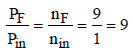7. In dilute aqueous H2SO4, the complex diaquodioxalatoferrate(II) is oxidized by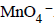. For this reaction, the ratio of the rate of change of [H+] to the rate of change of

Ans: 8

Solution :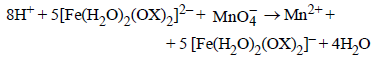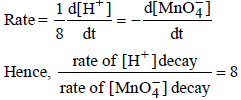Offer running on EduRev: Apply code STAYHOME200 to get INR 200 off on our premium plan EduRev Infinity!

69 docs|36 tests

,

,

,

,

,

,

,

,

,

,

,

,

,

,

,

,

,

,

,

,

,

,

,

,

;